# Krull-Remak-Schmidt theorem

Jump to: navigation, search

A series of propositions concerning the relationship between direct decompositions of a group or a ring. The lattice-theoretical version of the result is known as Ore's theorem (see Modular lattice). For a groupwith an arbitrary system of operators, one has the following theorem of Schmidt (R. Remak proved the result for finite groups , and W. Krull — for rings ): Ifadmits a principal series, then any two decompositions ofas a direct product with indecomposable factors are centrally isomorphic, i.e. there is a one-to-one correspondence between the sets of factors of each of the decompositions, and, ifand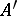are corresponding factors, there exists an isomorphism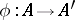such that, for each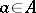,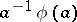lies in the centre of(, see also ). Schmidt's theorem, as a theorem for groups with operators is, in particular, valid for modules over any ring. However, a moduleis indecomposable if its endomorphism ring is local (see Local ring), and under certain restrictions (e.g. ifis a module of finite length) the converse is also true. In this connection, the Krull–Remak–Schmidt theorem for modules may be formulated as follows: Two decompositionswhere the endomorphism rings of the modulesand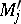are local, are isomorphic. Moreover, each term in one of the decompositions may be replaced by some term of the other. In some cases this replacement may also be done for an infinite set of terms. A category-theoretic approach has been developed to investigate questions connected with the Krull–Remak–Schmidt theorem; it uses the category of submodules of direct sums of the modules in question.